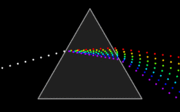#Drama! Intrigue! Geekiness!

## September 23, 2007

### No need of a hint this time..

Connect

2.Many apologies for the comment rejection mess. Special thanks to “Prasad the spam bot” for posting multiple comments, which lead us to the root of the problem.

Cracked by: srikanth , udupendra , Poornima , 2ndratequizzer , pratap , ramsaravana , uma , Sharath , Prasad , piezocake , Abhishek , prasanth , alephnull , Shashank , yaksha

1. Is a painting by William Blake. Its called Newton.
2. Dispersion of white light through a prism. One of the many things Newton discovered.
3. Apple’s Newton PDA/message pad. Its name is a tribute to Sir Issac Newton.

So, like all of you have guessed, the connect is Sir Issac Newton.

### 23 Responses to “No need of a hint this time..”

1. srikanth
 Newton 1) Newton, by WIlliam Blake 2) The concept that white light could be split into components was discovered/studied by Newton 3) The Apple Newton PDA

2. udupendraIsaac Newton 1. Newton as depicted by William Blake 2. One of the many things he is known for… 3. Apple's PDA

3. Poornima
 1: William Blake's Newton; Newton is depicted as a 'divine geometer' 2: Newton's refraction of light by a glass prism 3: The Apple Newton MessagePad connect: Newton

4.  Newton. The prism experiment, Apple's Newton, the forst pda, waaaaaaaaay ahead of its time it was…..but the first pic I dunno.

5.  Newton. The prism experiment, Apple's Newton (the first pda…waaay ahead of its time) Dunno the first pic.

6. pratap
 Newton

7.  the third pic is the PDA from Apple called Newton.. The second pic is  diffraction of light by Prism.. First is a pic of Newton… Newton explained the diffraction phenomenon

8.  pic 1: the painting “Newton” by William Blake, where Newton has been depicted as divine geometer. pic2: prism refracting the white light- Isaac Newton was the first to understand the colours and the rainbow phenomenon.he conducted a series of experiments called ’celebrated phenomenon of colors’ using prisms. pic3: The Apple Newton messagepad 100.It is an early line of personal digital assistants developed and marketed by Apple.The name was an allusion to Issac Newton’s apple. connect: Newton!!!

9. Sharath
 The connection seems to be Newton. Pic 1 : a painting of Sir Issac Newton. Pic 2: Newton was the first person to say white light is comprised of 7 different colors with his prism experiments. Pic 3:Apple Newton is an early PDA from the APPLE stable.

 Connect: Isaac Newton. 1 is "Newton," by William Blake,where Newton is depicted as a 'divine geometer' 2 shows decomposition of white light by a prism, which Newton discovered 3 is the Apple Newton

 1 is the paiting "Newton" by William Blake where Newton is depicted as 'the divine geometer' 2 shows the decomposition of white light by a prism, which Newton discovered 3 is the Apple Newton

 1 is the paiting "Newton" by William Blake where Newton is depicted as 'the divine geometer' 2 shows the decomposition of white light by a prism, which Newton discovered 3 is the Apple Newton

 1 is the painting "Newton" by William Blake where Newton is depicted as 'the divine geometer' 2 shows the decomposition of white light by a prism, which Newton discovered 3 is the Apple Newton

15. piezocake
 Newton.

16. Abhishek1)Painting of Newton by Blake 2)Newton's prism3)The Apple Newton MessagePad Connect : Isaac newton

17. Abhishek1)William Blake's "Isaac Newton" 2)Newton's Prism 3)The Apple Newton MessagePad Connect : Isaac newton

18. prasanthAll of them are connected through Newton 1) Newton's painting by blake 2) The concept of splitting white light into 7 colours 3) the apple newton, a Pda

19. alephnull
 Isaac Newton. Picture by Blake, the theory of optics that Newton elucidated and the ill-fated Apple Newton.

20. ShashankIssac Newton. 1. ?? 2. Newton found out Prism decomposes white light into visible spectrum. 3. Apple Newton btw, why doesn't your site accept all my comments?? I can't see my yesterday's answer :(

21. yaksha1>[wiki rip] "Newton," by William Blake; here, Newton is depicted as a 'divine geometer' [/wiki rip] 2>Splitting of white light through a prism 3>apple message pad running newton os connect is Issac Newton

22. ShashankIssac Newton 1. ?? 2. Issac Newton showed that white light is made up of 7 colors 3. Apple Newton btw, should i use xp+ie to post answers? if i post using ubuntu+ff or xp+ff, answers are not accepted!